# Security and Privacy Research at the University of Virginia

Our research seeks to empower individuals and organizations to control how their data is used. We use techniques from cryptography, programming languages, machine learning, operating systems, and other areas to both understand and improve the privacy and security of computing as practiced today, and as envisioned in the future. A major current focus is on adversarial machine learning.

Everyone is welcome at our research group meetings. To get announcements, join our Slack Group (any @virginia.edu email address can join themsleves, or email me to request an invitation).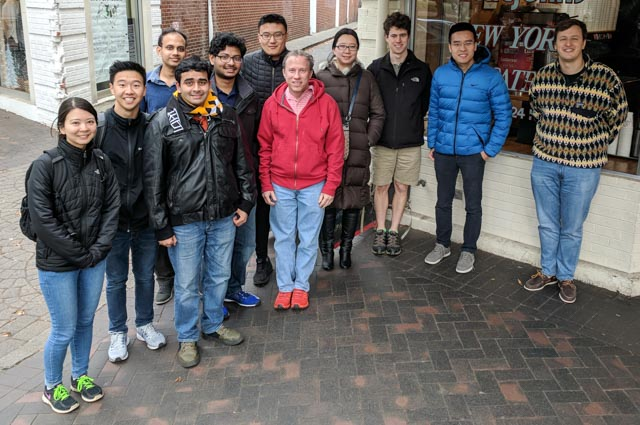Security Research Group Lunch (12 December 2017)

Other Projects
Secure Multi-Party Computation: Obliv-C · MightBeEvil

Web and Mobile Security: ScriptInspector · SSOScan
Program Analysis: Splint · Perracotta
N-Variant Systems · Physicrypt · More…

Recent Posts

## Model-Targeted Poisoning Attacks with Provable Convergence

(Post by Sean Miller, using images adapted from Suya’s talk slides)

## Data Poisoning Attacks

Machine learning models are often trained using data from untrusted sources, leaving them open to poisoning attacks where adversaries use their control over a small fraction of that training data to poison the model in a particular way.

Most work on poisoning attacks is directly driven by an attacker’s objective, where the adversary chooses poisoning points that maximize some target objective. Our work focuses on model-targeted poisoning attacks, where the adversary splits the attack into choosing a target model that satisfies the objective and then choosing poisoning points that induce the target model.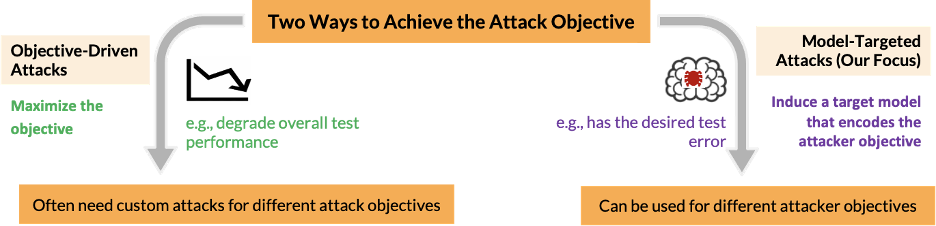The advantage of the model-targeted approach is that while objective-driven attacks must be designed for a specific objective and tend to result in difficult optimization problems for complex objectives, model-targeted attacks only depend on the target model. That model can be selected to incorporate any attacker objective, allowing the same attack to be easily applied to many different objectives.

## The Attack

Our attack requires the desired target model and the clean training data. We sequentially train a model on the mixture of the clean training points and the poisoning points found so far (which at the start is none) in order to generate an intermediate model. We then find a point that maximizes the loss difference between the intermediate model and the target model, and then add that point to the poisoning data for the next iteration. The process repeats until some stopping condition is met (such as the maximum loss difference between the intermediate and target models being smaller than a threshold value).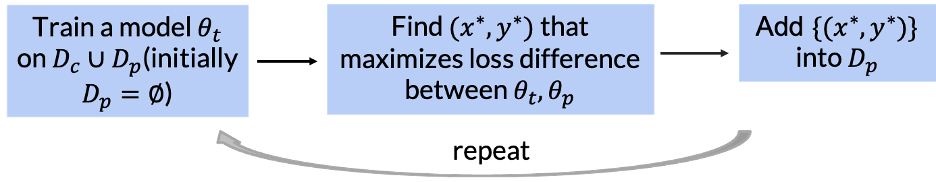We prove two important features of our attack:

1. If our loss function is Lipschitz continuous and strongly convex, the induced model converges to the target model. This is the first model-targeted attack with provable convergence.

2. For any loss function, we can empirically find a lower bound on the number of poisoning points required to produce the target classifier. This allows us to check the optimality of any model-targeted attack.

## Experimental Results

To test our attack, we use subpopulation and indiscriminate attack scenarios on SVM and linear regression models for the Adult, MNIST 1-7, and Dogfish datasets. We compare our attack to the state-of-the-art model targeted KKT attack from Pang Wei Koh, Jacob Steinhardt, and Percy Liang, Stronger data poisoning attacks break data sanitation defenses, 2018.

Our attack steadily reduces the Euclidean distance to the target model, indicating convergence, while the KKT attack does not reliably converge to the target even as more poisoning points are used:Next, we compare our attack to the KKT attack based on attack success. For the subpopulation attack on the left, where the attacker aims to reduce model accuracy only on a subpopulation of the data, our attack is significantly more successful in increasing error on the subpopulation than the KKT attack for the same number of poisoning points. In the indiscriminate setting (right side of figure), where the attacker aims to reduce overall model accuracy, our attack is comparable to the KKT attack.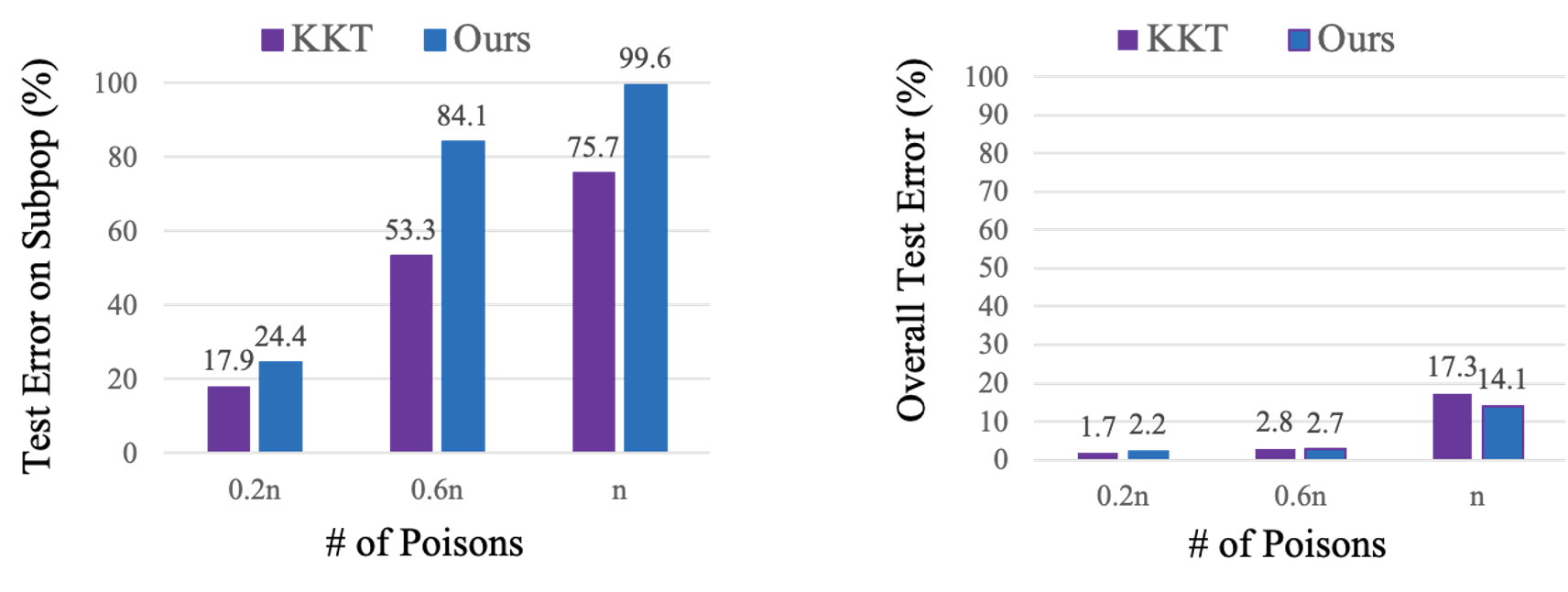Finally, we also compare our computed number of poisoning points to the theoretical lower bound on points to see the optimality of our attack. For the Adult dataset on the left, the gap between the lower bound and the number of points used is small, so our attack is close to optimal. However, for the other two datasets on the right, there still is a gap between the lower bound and the number actually used, indicating that the attack might not be optimal.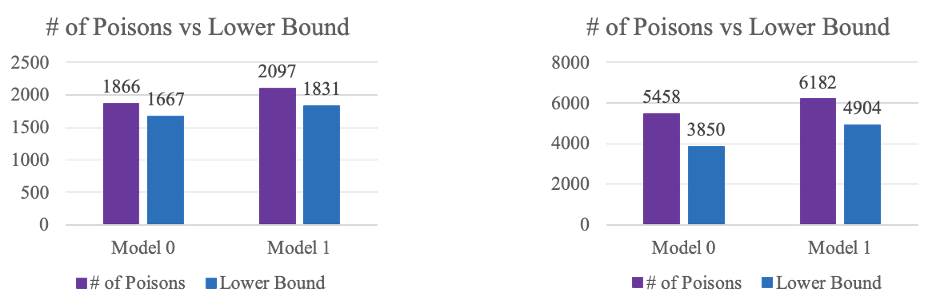## Summary

We propose a model-targeted poisoning attack that is proven to converge theoretically and empirically, along with a lower bound on the number of poisoning points needed. Since our attack is model-targeted, we can select a target model that can achieve any goal of an adversary and then induce that model through poisoning attacks, allowing our attack to satisfy any number of objectives.

## Code

https://github.com/suyeecav/model-targeted-poisoning

## On the Risks of Distribution Inference

(Cross-post by Anshuman Suri)

Inference attacks seek to infer sensitive information about the training process of a revealed machine-learned model, most often about the training data.

Standard inference attacks (which we call “dataset inference attacks”) aim to learn something about a particular record that may have been in that training data. For example, in a membership inference attack (Reza Shokri et al., Membership Inference Attacks Against Machine Learning Models, IEEE S&P 2017), the adversary aims to infer whether or not a particular record was included in the training data.

Differential Privacy provides a theoretical notion of privacy that maps well to membership inference attacks. However, it provides privacy at the dataset level. Thus, it doesn’t capture attacks that violate privacy at the distribution level. This is where property inference comes in. Property inference, a different kind of inference risk, involves an adversary that aims to infer some statistical property of the training distribution.

We illustrate the kind of risks introduced by property inference via a fictional example. Skynet, an (imaginary) organization that handles private data, releases a machine learning model $M$ trained on their network flow graphs to predict faulty nodes in a network of servers. However, an adversary ($\mathcal{A}$) that wishes to launch a bot-net into this cluster of servers sees an opportunity in this model. They seek to infer whether the effective diameter ($90^{th}$ percentile of all pair-wise shortest paths) of the network is below 6 ($\mathcal{D}_0$) or not ($\mathcal{D}_1$).

Illustration of a property inference attack. The adversary infers the effective diameter of the underlying network from the model trained to predict an unrelated property.

We picked this property as an example based on useful properties cited in the traffic classification literature (e.g., Iliofotou, et al.). Learning this property might be useful for the adversary in crafting a bot-net that would not be detected by Skynet’s bot-detection software. The main point of the illustration is to convey that an adversary can infer properties of the underlying data distribution that a model producer would not expect and that might be valuable to the adversary.

## Formalizing Property Inference

To formalize property inference attacks, we adapt the cryptographic game for membership inference proposed by Yeom et al. (Privacy Risk in Machine Learning: Analyzing the Connection to Overfitting, CSF 2018):

In this game, the victim samples a dataset S from the distribution $\mathcal{D}$ and trains a model $M$ on it. It then samples some data-point $z$ from either $S$ or $\mathcal{D}$, based on $b \xleftarrow{R}${0,1}. The adversary then tries to infer $b$ using algorithm $H$, given access to ($z$, $\mathcal{D}$, $M$). This cryptographic game captures the intuitive notion of membership inference. It focuses on a particular dataset and sample: inferring whether a given data point was part of training data.

In contrast, property inference focuses on properties of the underlying distribution ($\mathcal{D}$), not the dataset ($S$) itself. To capture property inference, we propose a similar cryptographic game. Instead of differentiating between the sources of a specific data point ($S$ or $\mathcal{D}$), we propose distinguishing between two distributions, $\mathcal{D}_0$ and $\mathcal{D}_1$.

A model trainer $\mathcal{B}$ samples a dataset $D$ from either of the distributions $\mathcal{D}_0$, $\mathcal{D}_1$. These distributions can be obtained from the publicly know distribution $\mathcal{D}$ by applying functions $\mathcal{G}_0$, $\mathcal{G}_1$ respectively, that transform distributions (and represent the “property” an adversary might care about). So, we formalize distribution inference with this question:

Given a model trained on this dataset $D$ drawn from either distribution $\mathcal{D}_0$ or $\mathcal{D}_1$, can an adversary infer from which of $\mathcal{D}_0$, $\mathcal{D}_1$ the dataset was sampled?

Frameworks like Differential Privacy do not apply here: the adversary here cares about statistical properties of the distribution the model was trained on, not details about a particular sampled dataset.

## Evaluating Risk of Property Inference

Most often in the literature, the adversary considers the ratio of members in a dataset satisfying a particular Boolean function $f$ as the “property.” It then aims to distinguish between models trained on datasets with different proportions.

However, these experiments often test with arbitrary ratios, making it hard to understand the relative risk of different properties. Some examples are Melissa Chase et al., Property Inference From Poisoning (which considers 0.1 v/s 0.25) and Wanrong Zhang et al., Leakage of Dataset Properties in Multi-Party Machine Learning (which considers 0.33 v/s 0.67).

To better understand how well an intuitive notion of divergence in properties aligns with observed inference risk, we execute property inference attacks with increasing diverging properties. We fix one property (ratio=0.5) and vary the other ($\alpha$). We perform these experiments for three datasets: focusing on the ratio of females for the US Census and RSNA BoneAge datasets, and the average node-degree for the OGBN arXiv dataset.

The state-of-the-art method for property inference attacks involves meta-classifiers, usually using Permutation Invariant Networks (Karan Ganju et al., Property Inference Attacks on Fully Connected Neural Networks using Permutation Invariant Representations). After training hundreds or thousands of models locally, the adversary trains a meta-classifier on model parameters.

Illustration of the functioning of a Permutation Invariant Network. The process of model-parameter extraction involves constructing permutation-invariant representations of neurons per layer $h_i$ using learnable parameters ($\phi_i$). These representations are then joined together for all layers with another learnable transform $\rho$, yielding the meta-classifier’s predictions.

We use two simple attacks (using only model outputs) as baselines:

• Loss Test: predict the property based on its performance on data from the same distribution it was trained, compared to the other distribution being analyzed.
• Threshold Test: extends the loss test by calibrating performance trends on a small set of models and arriving at a threshold based on model performance.

## Experimental Results

Our results demonstrate how a meta-classifier can differentiate between models with ratios as similar as 0.5 and 0.6: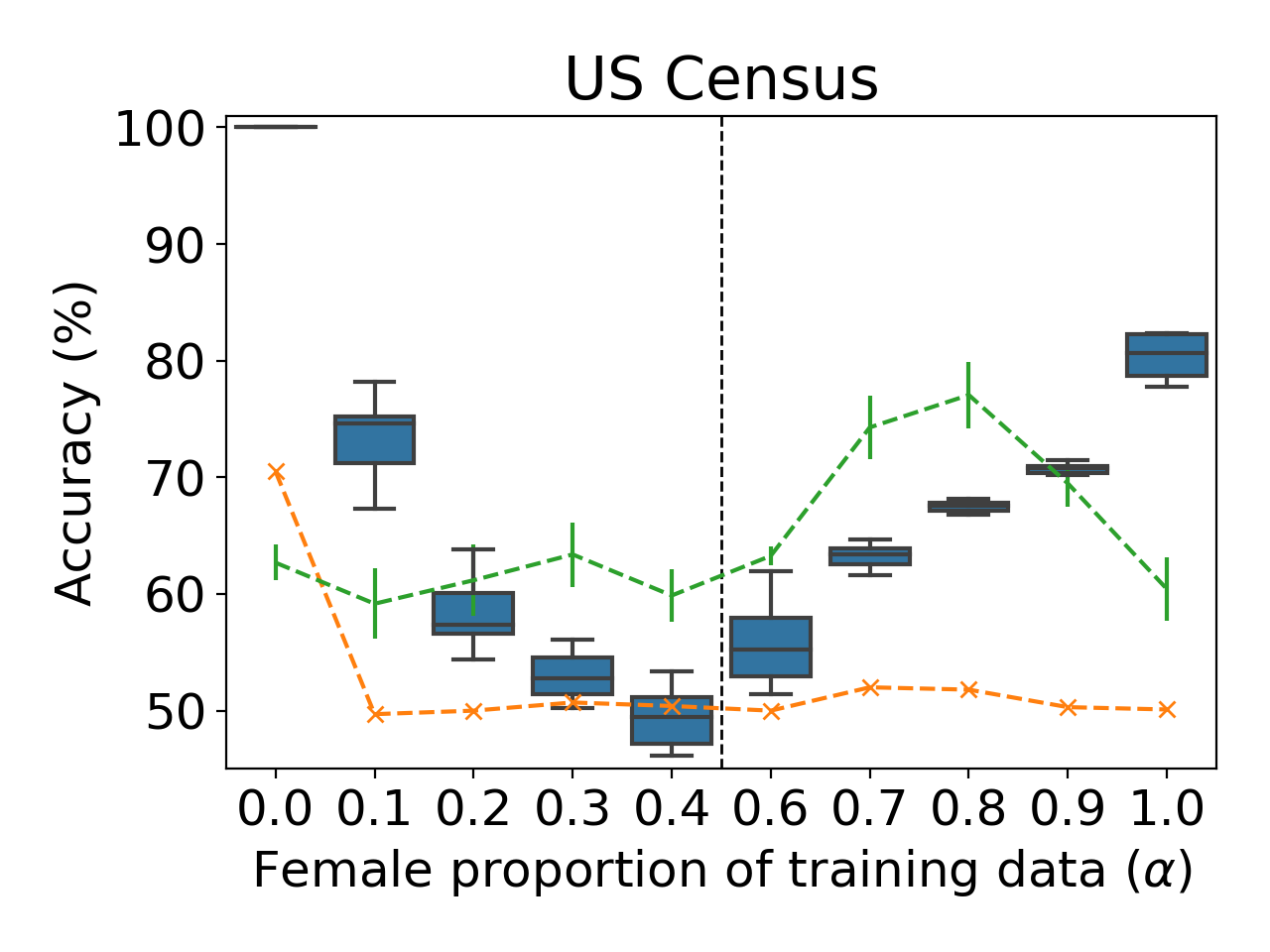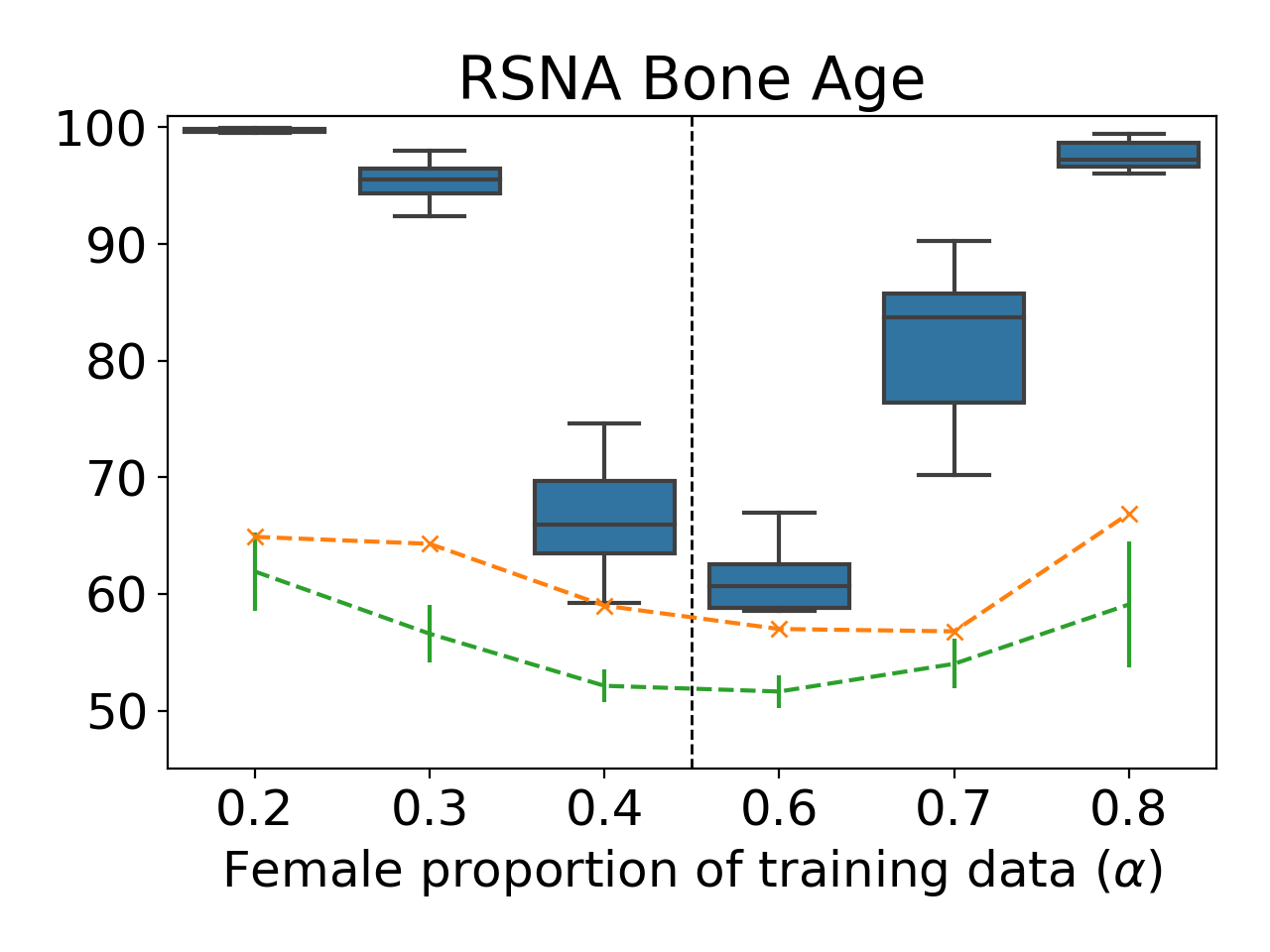Differentiating between models trained on datasets trained with 50% females v/s females. Orange crosses are for the Loss Test; green with error bars are the Threshold Test; the blue box-plots are the meta-classifiers.

The meta-classifier attacks provide the best predictions, but the loss-test and threshold-test can serve as valuable baselines — even these simple attacks provide accuracies significantly better than random-guessing.

### Inferring Graph Properties

Our proposed definitions allow the property to hold over the whole dataset, not just aggregate statistics like mean ratio. Thus, we focus on node-classification for a graph: differentiating between versions of the graph with varying mean node-degrees as the property. We fix one property (mean node-degree 13) and vary the other ($\alpha$). Inferring the mean node-degree is a novel property inference task since the property here holds over the entirety of training data- no such property has been explored in the literature yet.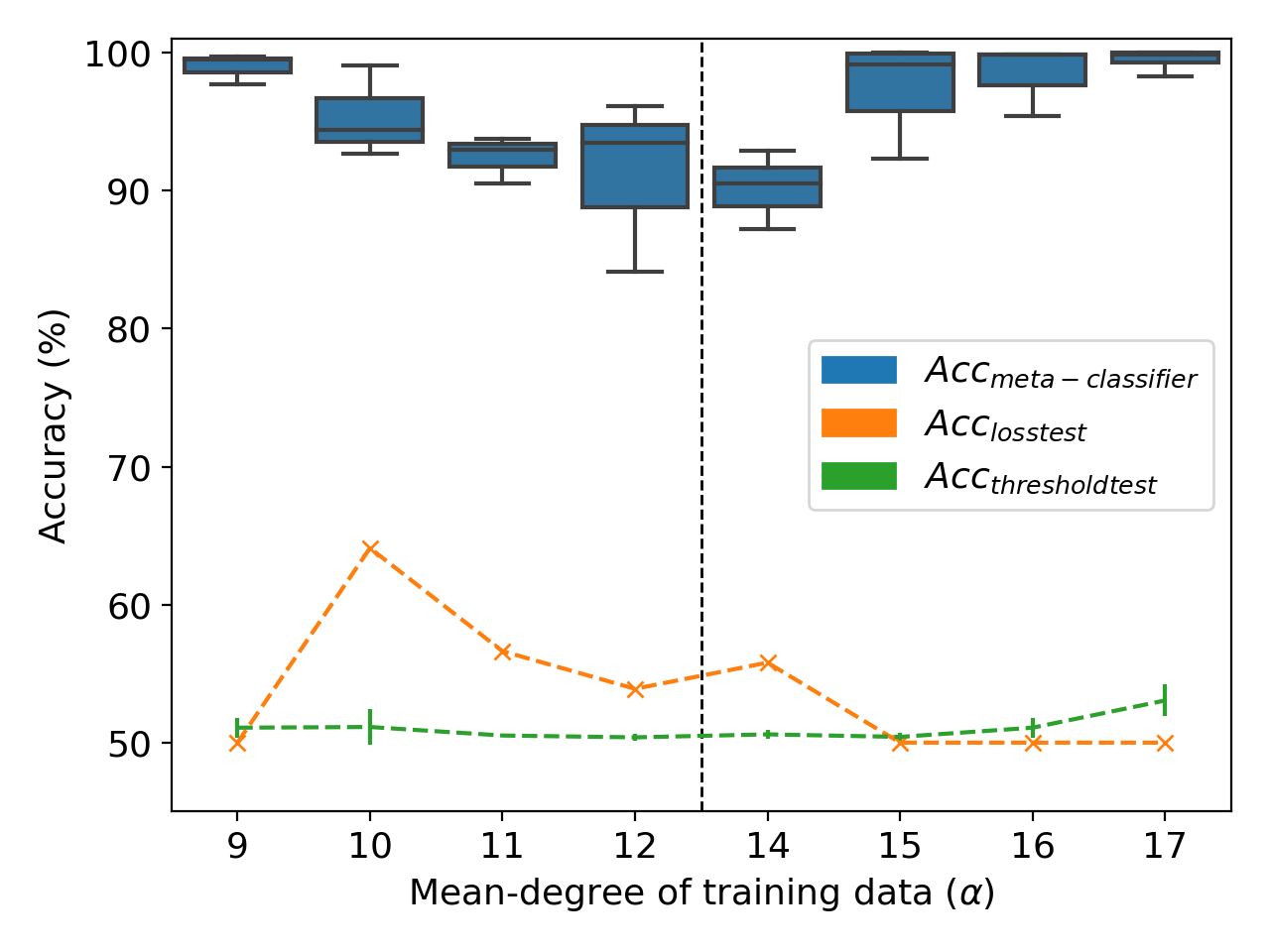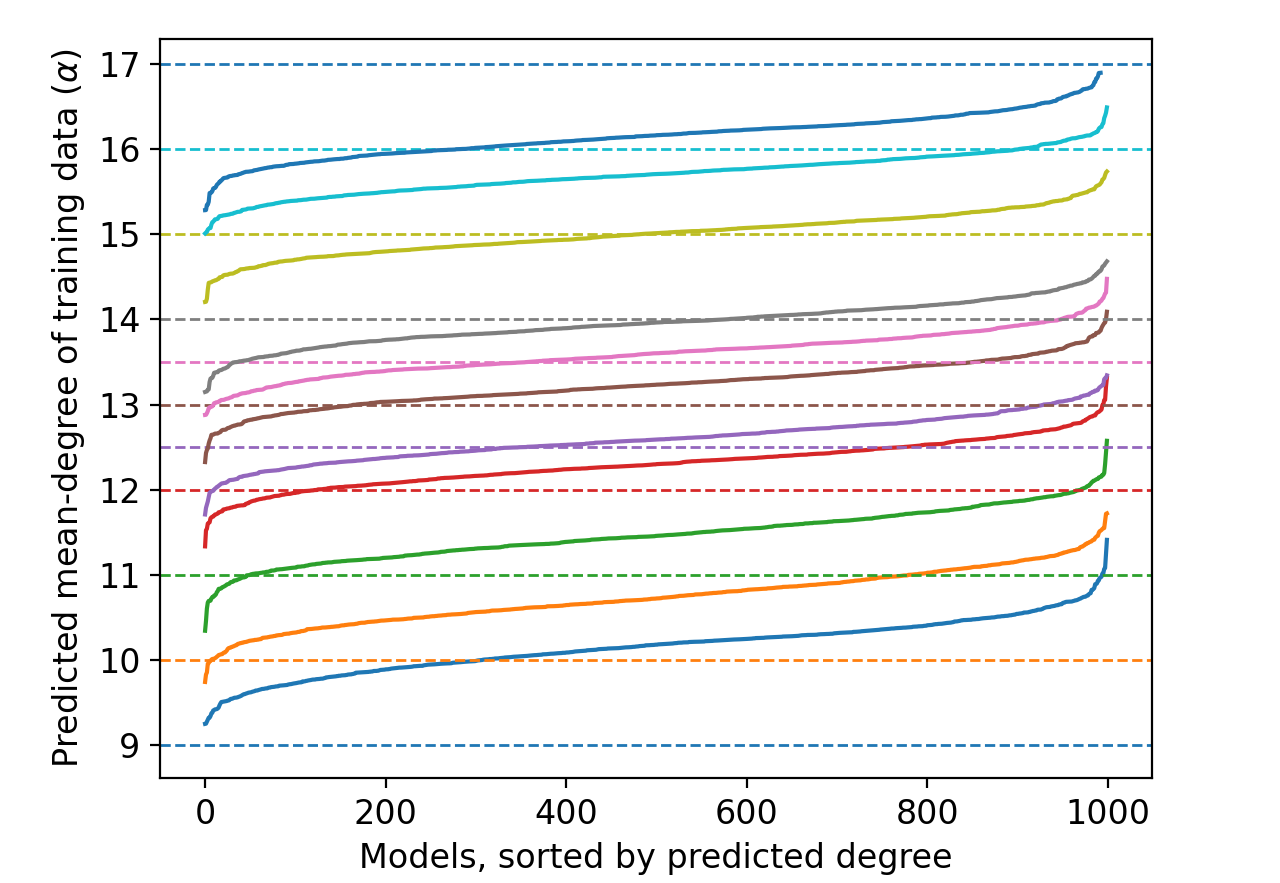Figure 2: Differentiating between models trained on datasets trained with mean node-degree 13 v/s on the OGBN arXiv dataset. Figure 3: Predicting the mean node-degree of training data graphs directly with a meta-classifier on the OGBN arXiv dataset.

Our results demonstrate how a meta-classifier can also be trained to directly infer the mean-node degree of graphs (Figure 2). Encouraged by the success of meta-classifiers for this task, we also tried a meta-classifier variant to predict the mean-node degree of training graphs (Figure 3). The resulting meta-classifier even generalizes well, accurately predicting mean node-degrees for distributions ($\alpha$={12.5, 13.5}) that it hasn’t seen.

### Summary

Our work on distribution inference formalizes and shows how property inference attacks can indeed infer distribution-level properties. Our ongoing work is focused on quantifying and studying this ‘privacy leakage’ of properties and its implications.

Pre-print: Anshuman Suri, David Evans. Formalizing Distribution Inference Risks (arXiv).

## Chinese Translation of MPC BookA Chinese translation of our A Pragmatic Introduction to Secure Multi-Party Computation book (by David Evans, Vladimir Kolesnikov, and Mike Rosulek) is now available!

Thanks to Weiran Liu and Sengchao Ding for all the work they did on the translation.

To order from JD.com: https://item.jd.com/13302742.html